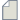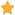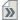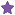###Author Topic: some questions about MPSH  (Read 4758 times)

0 Members and 1 Guest are viewing this topic.

####Roc

• QuantumATK Guru
•• Posts: 104
• Reputation: 0« on: December 27, 2008, 09:52 »
Dear everyone,

In this tutorial analyzing the Li-H2-Li two-probe system, a part of one script is that,

# Calculate HOMO and LUMO eigenstates for the H atoms in the two-probe system
eigenstates = calculateProjectedHamiltonianEigenstates(
scf, projection_atoms = [3,4],
quantum_numbers = [0,1]
)

For other two-probe systems, how to decide the "Quantum Numbers " in VNL when I calculate Projected Hamiltonian eigenstates to obtain the HOMO、LUMO、HOMO-N and LUMO+N

Thanks!

####Nordland

• QuantumATK Staff
• Supreme QuantumATK Wizard
•• Posts: 812
• Reputation: 18##### Re: some questions about MPSH
« Reply #1 on: December 27, 2008, 19:11 »
The way I would do it, is not automated, but I will use the function calculateProjectedHamiltonianEnergySpectrum - you can see the documentation here.

From that you will find the energy spectrum, and you can identify the quantum number for the homo-lumo from the the energies since zero is the fermi energy.
You will the find the HOMO as the first quantum number below zero ( in order words the last negative one) and the LUMO as the first above zero (in order words the first positive energy). Then you can define N as you want since you will have the quantum numbers for both the HOMO and LUMO.

But it is only a suggestion.
« Last Edit: December 28, 2008, 09:18 by Nordland »

####Roc

• QuantumATK Guru
•• Posts: 104
• Reputation: 0##### Re: some questions about MPSH
« Reply #2 on: December 28, 2008, 01:46 »
Thank you very much, Nordland ! I see.